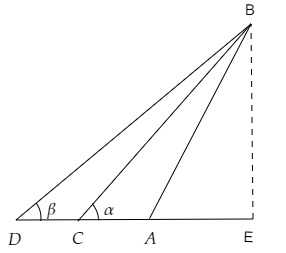# A tree standing on a horizontal plane is leaning towards east. At two points situated at distances $a$ and $b$ exactly due west on it, the angles of elevation of the top are respectively $\alpha$ and $\beta$. Prove that the height of the top from the ground is $\frac{(b-a) \tan \alpha \tan \beta}{\tan \alpha-\tan \beta}$

Given:

A tree standing on a horizontal plane is leaning towards east. At two points situated at distances $a$ and $b$ exactly due west on it, the angles of elevation of the top are respectively $\alpha$ and $\beta$.

To do:

We have to prove that the height of the top from the ground is $\frac{(b-a) \tan \alpha \tan \beta}{\tan \alpha-\tan \beta}$.

Solution:Let $AB$ be the tree and $C$ and $D$ are two points situated at distances $a$ and $b$ exactly due west on it.

Draw a perpendicular to the ground from the top of the tree.

From the figure,

$\angle BCE=\alpha, \angle BDE=\beta$

Let the height of the perpendicular be $h$, the distance between $A$ and $E$ be $x$.

In right $\Delta \mathrm{BCE}$,

$\tan \alpha=\frac{BE}{CE}$

$=\frac{h}{x+a}$

$x+a=\frac{h}{\tan \alpha}$

$x=\frac{h}{\tan \alpha}-a$..........(i)

Similarly,

In right $\Delta \mathrm{BDE}$,

$\tan \beta=\frac{BE}{DE}$

$=\frac{h}{x+b}$

$h=(x+b)\tan \beta$

$x=\frac{h}{\tan \beta}-b$............(ii)

From (i) and (ii), we get,

$\frac{h}{\tan \alpha}-a=\frac{h}{\tan \beta}-b$

$\Rightarrow h(\frac{1}{\tan \alpha}-\frac{1}{\tan \beta})=a-b$

$h(\frac{\tan \beta-\tan \alpha}{\tan \alpha \tan\ \beta})=a-b$

$h=\frac{(b-a) \tan \alpha \tan \beta}{\tan \alpha-\tan \beta}$

Hence proved.

Updated on: 10-Oct-2022

37 Views